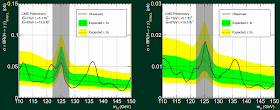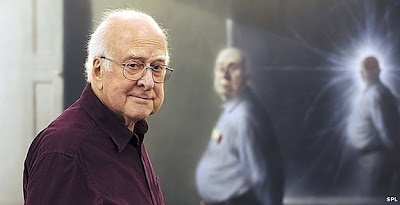## Monday, July 01, 2013

### CMS: $$2.93\sigma$$ hint of a second Higgs boson at $$136.5\GeV$$

Today's sensation is tomorrow's calibration. Or: Today's discovery is tomorrow's background. Ladies and Gentlemen, it's my pleasure to tell you that we are finally living in the tomorrow in the sense of these proverbs. CMS has finally published a paper that incorporates the $$125$$-$$126\GeV$$ Higgs boson into the background.
Properties of the observed Higgs-like resonance decaying into two photons (CMS PAS HIG-13-016)
ATLAS and CMS are doing a superb precision work. One of the consequences is that their papers have been found to agree with the Standard Model immensely well. In fact, we haven't seen virtually any $$2\sigma$$ excesses. One could argue that the number of false positives in other experiments than ATLAS and CMS – bumps that would fill many physicists with a "hope" that turned out to be unjustified – was much higher than 5% and the reason was that these experimenters were just sloppier than ATLAS and CMS.

But neither new physics nor flukes can be denied and confined indefinitely. The paper above which was released today is an example.

They have studied how many Higgs bosons are produced that decay into two photons, $$h\to \gamma\gamma$$, and the final number agrees with the predictions of the Standard Model within one sigma. This invalidates some "hopes" that the LHC had seen way too many diphoton decays a year ago or so. This particular new paper can't exclude, based on the spin-related measurements of the diphoton processes only, that the Higgs particle is a graviton-style spin-two particle but there are other experimental and especially theoretical ways to de facto exclude the possibility.

But while the old false hope has been killed, there's a new one. They made a search for another Higgs-like resonance aside from the $$125$$-$$126\GeV$$ Higgs boson that has already been discovered. And here is the main graph showing the results:It's Figure 3 – in the paper, you also see a combination of these two graphs at the top. The left graph requires the Higgs to come from the vector-boson fusion while the right graph above is from the vector-boson associated production only. If my understanding is right, these two graphs should visualize two totally disjoint sets of proton-proton collisions.

What you see on the left graph is a rather clear excess near $$m_H=136.5\GeV$$, as the paper quantifies the location. We're told that the local significance of this extra peak is $$2.73\sigma$$. That's far from a discovery but it's a lot. The right graph should come from different events but it also has a $$2.15\sigma$$ excess, as they quantify it. What is the mass of the would-be new particle that would follow from this second bump if it were real? Believe me or not, it is$m_H = 136.5\GeV.$ Quite a coincidence, right? If you need to know,$\sqrt{2.73^2+2.15^2} = 3.47.$ In some sense, unless I am making a mistake, the total local significance of the excess is a brutally tantalizing $$3.5\sigma$$. That's a lot. Well, their own combination of both parts of Figure 3, namely Figure 2, quotes the overall local significance as $$2.93\sigma$$. I can't explain you where the discrepancy comes from but we're near $$3\sigma$$, anyway. (Ulrich Ellwanger explained to me that the significance $$3.5\sigma$$ is an overestimate because the two components share a systematic error.)

Needless to say, minimal and rather minimal supersymmetric standard models of particle physics often predict that along with a Higgs boson below $$130\GeV$$, there should be another equally CP-even neutral Higgs boson above $$130\GeV$$. The other three Higgs bosons among the five God particles of the MSSM are two charged ones; and one CP-odd boson $$A$$.Only the left Higgs, one with the mass $$125$$-$$126\GeV$$, is clearly real at this point. The other Higgses with different masses, indicated by differently stuffed bellies, are rather likely to turn out to be just mirages.

In recent years, it has become popular to assume that the other Higgses predicted by supersymmetry is much heavier than the Higgs boson found almost exactly one year ago. But the possibility that their masses are really close hasn't really been excluded yet. Under a certain paradigm, it actually seems extremely natural for the two Higgses to be distributed quasisymmetrically with respect to the $$130\GeV$$ boundary between "light" and "heavy" Higgs bosons which supersymmetry likes to draw.

It may be a good idea to wait for the analogous paper by ATLAS (or is it already out?). If it provided us with some $$3.5\sigma$$ evidence as well, the local informal significance of this new peak could alredy surpass $$5\sigma$$. And that would start to be really interesting, well before the $$13\TeV$$ run that will begin in April 2015. It could be a good enough reason for Jester and others to buy some new underwear.

Fascinatingly enough, a $$3.5\sigma$$ excess at the precisely the same mass, $$136.5\GeV$$, seen in the analogous CMS diphoton graphs was being debated already one year ago (in the wake of the first official Higgs discovery) when a much (2.5 times) smaller dataset, $$5.1+5.3/{\rm fb}$$, was available. The significance should have dropped but it stayed the same.

Update: Pages 5-6 of some ATLAS presentation suggest that ATLAS has seen nothing over there. There's a nearby excess at $$141\GeV$$ or so but that's already significantly different, despite the fact that ATLAS tends to report the masses in the diphoton channel that are up to $$3\GeV$$ higher than those of CMS. But one needs $$4.5\GeV$$ here... ;-)

1.There's one important piece of evidence still missing though:

You making this \$100 bet with Jester at odds of 100:1 in your favour.

2.what is the excess once the "look elsewhere" effect has been taken into account?

3.Maybe the 2.93 sigma figure (combining both channels) *is* after the look-elsewhere effect is incorporated, therefore explaining why it's lower then my simple result 3.47 sigma. The wording is a bit ambiguous.

4.Θ³Σx² - ∂³Σx² - ΘΣJul 1, 2013, 9:17:00 PM

1/(alpha) GeV???? Fascinating! :). Note that the fine structure constant is also in the air with both values. Challenge: is the relation with alpha just a coincidence or something deeper? My conjecture, long ago, was that the higgs mass, understood as coupling, should provide M(H)=137=1/(alpha), and/or M(h)=1/(alpha(H))...Question for you Dr.Motl...Are a 127-128 GeV and/or an additional (neutral?) 137GeV good news for susy theories? ATLAS seemed to exclude Higgses above the one...If today were NOT 1 July I would feel strangely disappointed with the ATLAS team ;)... This is my contribuntion to the 1 July "party"... XD XD...

5.Wow, I didn't look at the mass. ;-)

6.alejandro riveroJul 2, 2013, 2:49:00 PM

I seriously hope that you are thinking in some unit system where proton mass is equal to one or some similar adjustment you forgot to tell. Because if not, you are just spreading fog.

7.I would like to observe that it is not enough to have the data, you need also all the megabytes of metadata which describe the boundaries and conditions of the experiment before you can filter the baby out of the bathwater. The reason there are 3000 people in these experiments (and maybe half of them scrambling to find new physics) is because of the complexity of the data . Just looking of the beam intersection in one event should convince you of this, where there are twenty or so events that have to be untangled in the same time "frame" .

If you do not have the meta data you will find lots of beautiful peaks : problems in the complex detectors with the thousands of elements can generate spurious peaks. These excite new or naive people for a while, but they come down to reality and understand that any peak has to be combed through the meta data before getting excited.

8.Anna, here's a brilliant, and very funny strategy for combating NSA wiretapping and data mining:

http://therealnews.com/t2/component/hwdvideoshare/viewvideo/76479/political-humor/nsa-wiretapping-public-service-announcement

9.Lubos,

Why no dark matter effect on the solar system?

http://arxiv.org/abs/1306.5534

Thanks

10.I seriously hope that you are
thinking in some unit system where proton mass is equal to one or some
similar adjustment you forgot to tell. Because if not, you are just